Latest Banking jobs   »   Quantitative Aptitude Quiz For SBI/IBPS PO...

Quantitative Aptitude Quiz For SBI/IBPS PO Mains 2021- 16th December

Q1. A diagram is given below. AC is the diameter of circle. AD || BC and ∠ACD=90° ∠ACB≤45°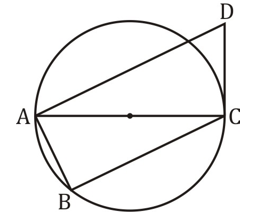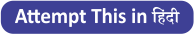Quantity II: – 45°
(a) Quantity I > Quantity II
(b) Quantity I < Quantity II
(c) Quantity I ≥ Quantity II
(d) Quantity I ≤ Quantity II
(e) Quantity I = Quantity II or No relation

Q2. Quantity I: ‘x’ : There is an interview conducted by HCL. Out of total applicants six are female. Probability of selecting two candidates such that at least one is male is 7/8. ‘x’ is total number of males.
Quantity II: ‘y’ : A, B and C together start a business. Ratio between investment of A and B is 15 : y while ratio between investment of B & C is 9 : 11. A left business after 8 months of starting of business and B left before 7 months of completion of year. Out of total profit of Rs. 2080, A got Rs. 900.
(a) Quantity I > Quantity II
(b) Quantity I < Quantity II
(c) Quantity I ≥ Quantity II
(d) Quantity I ≤ Quantity II
(e) Quantity I = Quantity II or No relation

Directions (3-6): The following questions are accompanied by three statements A, B and C. You have to determine which statement(s) is/are necessary/sufficient to answer the question.

Q3. Find the area of the shaded region?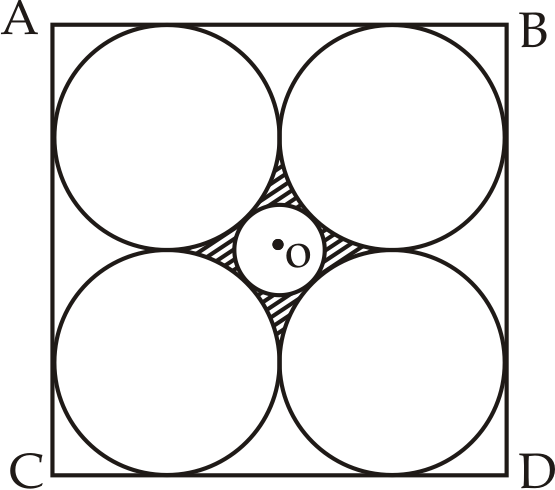A. Radius of bigger circle is given.
B. Diagonal of square ABCD is given.
C. Difference between area of bigger circle and area of smaller circle is given.
(a) Either A or B alone is sufficient to answer the question
(b) Either B or C alone is sufficient to answer the question
(c) Either A or C alone is sufficient to answer the question
(d) Any of A, B or C alone is sufficient to answer the question
(e) Either only A or B and C together is sufficient to answer the question

Q4. Find the surface area of cone?
A. Ratio between height and radius of cone is 8 : 7.
B. Radius of cone is half of the radius of hemisphere, which volume is 19404 cm³.
C. Radius of cone is 75% of radius of cylinder, which total surface area of 2640 cm³ and ratio between height and radius of cylinder is 8 : 7.
(a) Either A and B or B and C are sufficient to answer the question
(b) Either A and B or A and C are sufficient to answer the question
(c) Either A and C or B and C are sufficient to answer the question
(d) A, B and C together are sufficient to answer the question
(e) Either only A or B and C together are sufficient to answer the question

Q5. What will be 26 times of 26th term of Arithmetic progression (AP)?
A. If eight times of the 8th term of that A.P. is equal to 18th times the 18th term of that A.P.
B. If first term of AP is a prime number.
C. If difference between two terms in A.P. is an odd number.
(a) Only statement ‘A’ alone is sufficient to answer the question
(b) Only statement ‘B’ alone is sufficient to answer the question
(c) Only statement ‘C’ alone is sufficient to answer the question
(d) Any of two statements are sufficient to answer the question
(e) All three together are sufficient to answer the question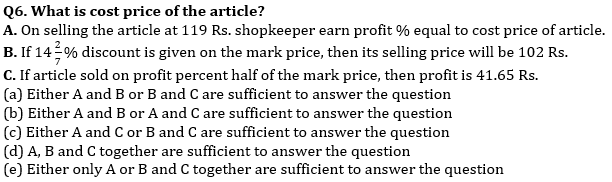Q7. Quantity I: ‘x’ : Two mutually perpendicular chords PQ and EF meet at a point S inside a circle. PS = 12 cm, SQ = 8 cm and FS = 6 cm. ‘x’ is the area of circle.
Quantity II: ‘y’ : In a circle, two parallel chords are drawn on opposite sides of diameter. Distance between these chords is 14cm. Length of one chord is 16cm while length of another chord is 12cm. ‘y’ is the area of circle
(a) Quantity I > Quantity II
(b) Quantity I < Quantity II
(c) Quantity I ≥ Quantity II
(d) Quantity I ≤ Quantity II
(e) Quantity I = Quantity II or No relation

Q8. Quantity I: ‘x’ :A dishonest shopkeeper sales his good by weighting 15% less and cheat his wholesaler by giving 15% less amount. ‘x’ is his approximate total profit % if he sells it at cost price.
Quantity II: ‘y’ : A dishonest milkman, add 20 litre water in pure milk, after selling half of mixture. He again added 20 liter of water in remaining mixture now milk and water ratio in mixture becomes 4 : 3. ‘y’ is his overall profit % on selling total mixture.
(a) Quantity I > Quantity II
(b) Quantity I < Quantity II
(c) Quantity I = Quantity II or No relation
(d) Quantity I ≥ Quantity II
(e) Quantity I ≤ Quantity II

Q9. Quantity I: ‘x’ : A and B can do a work in 24 days together, in which 40% of work is done by B. C who is 25% more efficient than A, do the same work alone in ‘x’ days.
Quantity II: ‘y’ : P, Q and S work for x, x+1 and x+2 days and their efficiency are in the ratio of x : x+1 : x+2 respectively. If P got 16 Rs for his work, then ‘y’ is the total money earned by all three in Rs.. (Compare Magnitude of x and y)
(a) Quantity I > Quantity II
(b) Quantity I ≥ Quantity II
(c) Quantity I = Quantity II or No relation
(d) Quantity I < Quantity II
(e) Quantity I ≤ Quantity II

Q10. Quantity I:’x’ -. Two circles are concentric with center ‘O’. Their radii are 8cm and 10cm respectively. ‘B’ and ‘C’ are the points of contact of two tangents drawn from bigger circle to smaller circle from point ‘A’ lying on bigger circle. ‘x’ is area of quadrilateral ABOC formed in cm²
Quantity II: – ‘y’ – The lengths of two parallel sides of a trapezium are 6 cm and 8 cm. If the height of the trapezium be 6 cm, then its area is ‘y’ cm²
(a) Quantity I > Quantity II
(b) Quantity I < Quantity II
(c) Quantity I ≥ Quantity II
(d) Quantity I ≤ Quantity II
(e) Quantity I = Quantity II or No relation

Solutions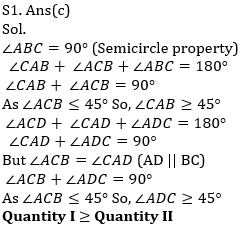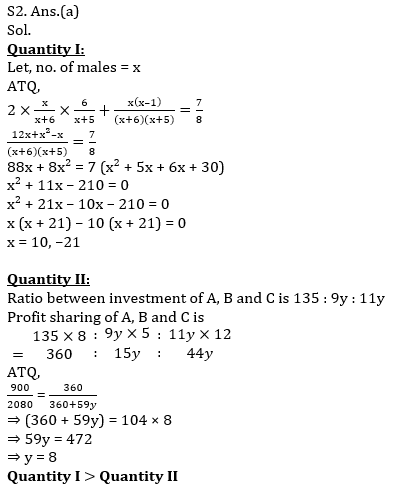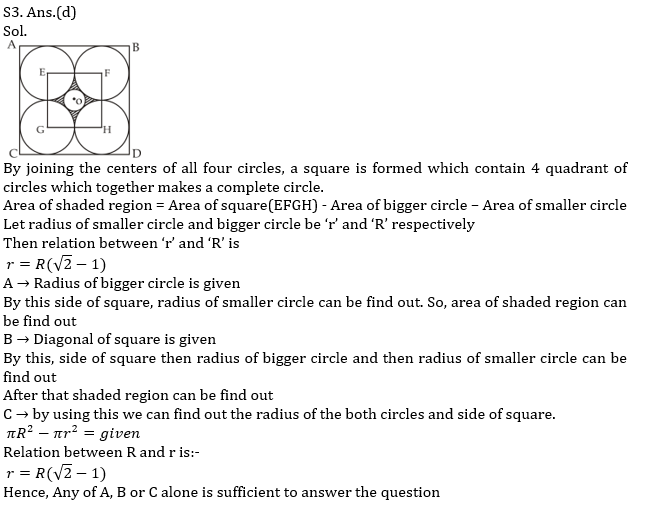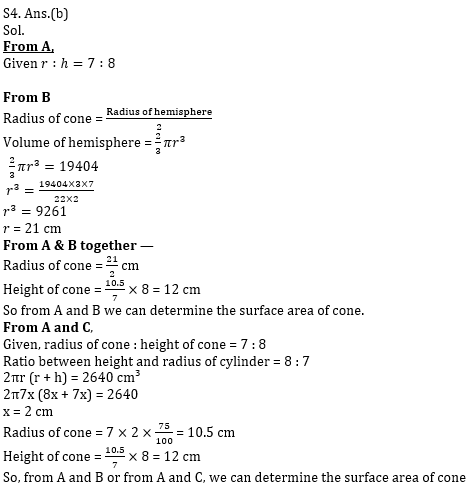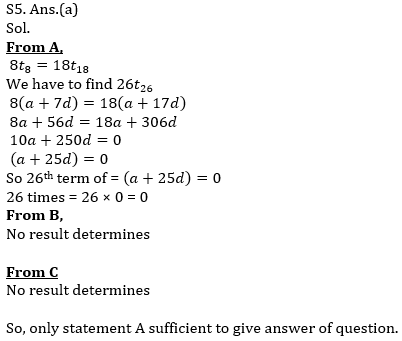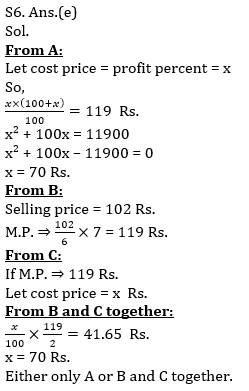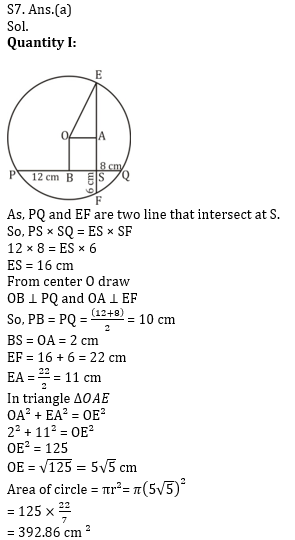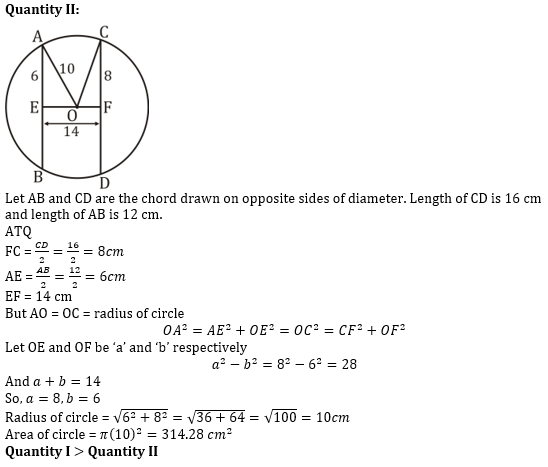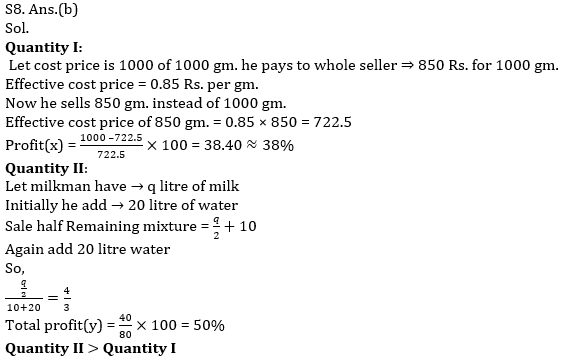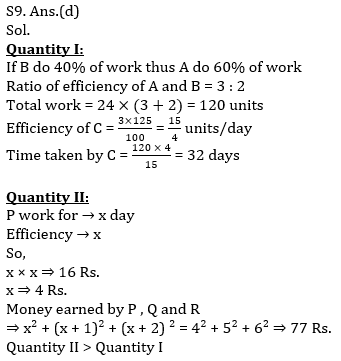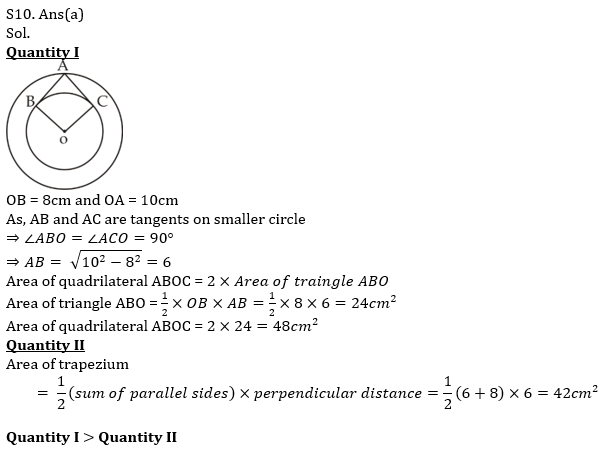×

Thank You, Your details have been submitted we will get back to you.Join India's largest learning destination

What You Will get ?

•Job Alerts
•Daily Quizzes
•Subject-Wise Quizzes
•Current Affairs
•Previous year question papers
•Doubt Solving session

ORJoin India's largest learning destination

What You Will get ?

•Job Alerts
•Daily Quizzes
•Subject-Wise Quizzes
•Current Affairs
•Previous year question papers
•Doubt Solving session

ORJoin India's largest learning destination

What You Will get ?

•Job Alerts
•Daily Quizzes
•Subject-Wise Quizzes
•Current Affairs
•Previous year question papers
•Doubt Solving session

Enter the email address associated with your account, and we'll email you an OTP to verify it's you.Join India's largest learning destination

What You Will get ?

•Job Alerts
•Daily Quizzes
•Subject-Wise Quizzes
•Current Affairs
•Previous year question papers
•Doubt Solving session

Enter OTP

Please enter the OTP sent to
/6

Did not recive OTP?

Resend in 60sJoin India's largest learning destination

What You Will get ?

•Job Alerts
•Daily Quizzes
•Subject-Wise Quizzes
•Current Affairs
•Previous year question papers
•Doubt Solving sessionJoin India's largest learning destination

What You Will get ?

•Job Alerts
•Daily Quizzes
•Subject-Wise Quizzes
•Current Affairs
•Previous year question papers
•Doubt Solving session

Almost there

+91Join India's largest learning destination

What You Will get ?

•Job Alerts
•Daily Quizzes
•Subject-Wise Quizzes
•Current Affairs
•Previous year question papers
•Doubt Solving session

Enter OTP

Please enter the OTP sent to Edit Number

Did not recive OTP?

Resend 60

By skipping this step you will not recieve any free content avalaible on adda247, also you will miss onto notification and job alerts

Are you sure you want to skip this step?

By skipping this step you will not recieve any free content avalaible on adda247, also you will miss onto notification and job alerts

Are you sure you want to skip this step?